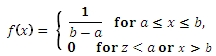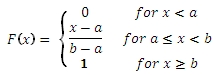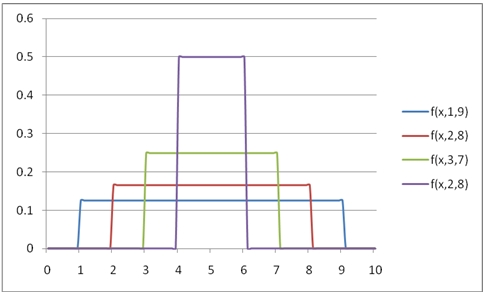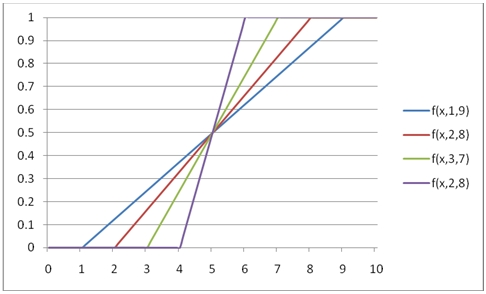# SQL Server uniform distribution function

UNIFORM

Updated: 31 July 2010

Use UNIFORM to calculate the probability density function or the lower cumulative distribution function of the uniform distribution.

The formula for the probability density function is:The lower cumulative distribution function is:Syntax
SELECT [wctStatistics].[wct].[UNIFORM] (
<@X, float,>
,<@Min, float,>
,<@Max, float,>
,<@Cumulative, bit,>)
Arguments
@X
is the value to be evaluated. @X is an expression of type float or of a type that implicitly converts to float
@Min
is the minimum value of the distribution. @Min is an expression of type float or of a type that implicitly converts to float
@Max
is the maximum value of the distribution. @Max is an expression of type float or of a type that implicitly converts to float
@Cumulative
is a logical value that determines if the probability density function ('False', 0) or the cumulative distribution function ('True', 1) is being calculated. @Cumulative is an expression of type bit or of a type that implicitly converts to bit
Return Types
float
Remarks
·         @Max must be greater than or equal to @Min (@Max > @Min).
Examples
Calculate the probability density function:
SELECT wct.UNIFORM(2,1,5,'False')
This produces the following result
----------------------
0.25

(1 row(s) affected)

You can use the SeriesFloat function from the XLeratorDB/math library to generate a dataset which can be pasted into EXCEL to generate a graph of the probability density function.
SELECT SeriesValue
,wct.UNIFORM(SeriesValue, 1, 9, 'False') as [f(x,1,9)]
,wct.UNIFORM(SeriesValue, 2, 8, 'False') as [f(x,2,8)]
,wct.UNIFORM(SeriesValue, 3, 7, 'False') as [f(x,3,7)]
,wct.UNIFORM(SeriesValue, 4, 6, 'False') as [f(x,2,8)]
FROM wctMath.wct.SeriesFloat(0,10,.1,NULL,NULL)
This is an EXCEL-generated graph of the resultsCalculate the lower cumulative distribution function:
SELECT wct.UNIFORM(3,1,5,'True')
This produces the following result
----------------------
0.5

(1 row(s) affected)

You can use the SeriesFloat function from the XLeratorDB/math library to generate a dataset which can be pasted into EXCEL to generate a graph of the cumulative distribution function.
SELECT SeriesValue
,wct.UNIFORM(SeriesValue, 1, 9, 'True') as [f(x,1,9)]
,wct.UNIFORM(SeriesValue, 2, 8, 'True') as [f(x,2,8)]
,wct.UNIFORM(SeriesValue, 3, 7, 'True') as [f(x,3,7)]
,wct.UNIFORM(SeriesValue, 4, 6, 'True') as [f(x,2,8)]
FROM wctMath.wct.SeriesFloat(0,10,.1,NULL,NULL)
This is an EXCEL-generated graph of the results### SupportCopyright 2008-2021 Westclintech LLC         Privacy Policy        Terms of Service